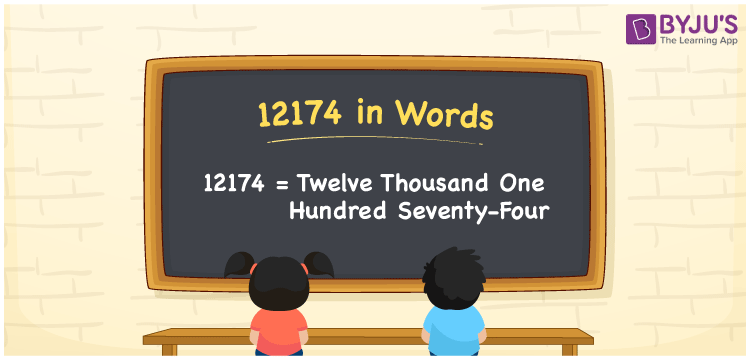# 12174 in words

12174 in words is written as Twelve Thousand One Hundred and Seventy Four. In 12174, 1 has a place value of ten thousand, 2 is in the place value of thousand, 1 is in the place value of hundred, 7 is in the place value of ten and 4 is in the place value of one. The article on Place Value gives more information. The number 12174 is used in expressions that relate to money, distance, social media views, and many more. For example, “The diameter of the planet Venus is seventy less than Twelve Thousand One Hundred and Seventy Four.”

 12174 in words Twelve Thousand One Hundred and Seventy Four. Twelve Thousand One Hundred and Seventy Four in Numbers 12174

## 12174 in English Words## How to Write 12174 in Words?

We can convert 12174 to words using a place value chart. The number 12174 has 5 digits, so let’s make a chart that shows the place value up to 5 digits.

 Ten thousand Thousands Hundreds Tens Ones 1 2 1 7 4

Thus, we can write the expanded form as:

1 × Ten thousand + 2 × Thousand + 1 × Hundred + 7 × Ten + 4 × One

= 1 × 10000 + 2 × 1000 + 1 × 100 + 7 × 10 + 4 × 1

= 12174.

= Twelve Thousand One Hundred and Seventy Four.

12174 is the natural number that is succeeded by 12173 and preceded by 12175.

12174 in words – Twelve Thousand One Hundred and Seventy Four.

Is 12174 an odd number? – No.

Is 12174 an even number? – Yes.

Is 12174 a perfect square number? – No.

Is 12174 a perfect cube number? – No.

Is 12174 a prime number? – No.

Is 12174 a composite number? – Yes.

## Solved Example

1. Write the number 12174 in expanded form

Solution: 1 x 10000 + 2 x 1000 + 1 x 100 + 7 x 10 + 4 x 1

We can write 12174 = 10000 + 2000 + 100 + 70 + 4

= 1 x 10000 + 2 x 1000 + 1 x 100 + 7 x 10 + 4 x 1.

## Frequently Asked Questions on 12174 in words

Q1

### How to write the number 12174 in words?

12174 in words is written as Twelve Thousand One Hundred and Seventy Four..
Q2

### Is 12174 a perfect square number?

No. 12174 is not a perfect square number.
Q3

### Is 12174 a prime number?

No. 12174 is not a prime number.